# Transportation Engineering: Location, Geometrics, and Drainage

A +2 percent grade on an arterial highway intersects with a -1 percent grade at station (535 + 24.24) at an elevation of 300 ft. if the design speed of the highway is65 mi/h, determine the stations and elevations of the BVC and EVC, the elevation of each 100-ft station, and the high point. Use L= KA.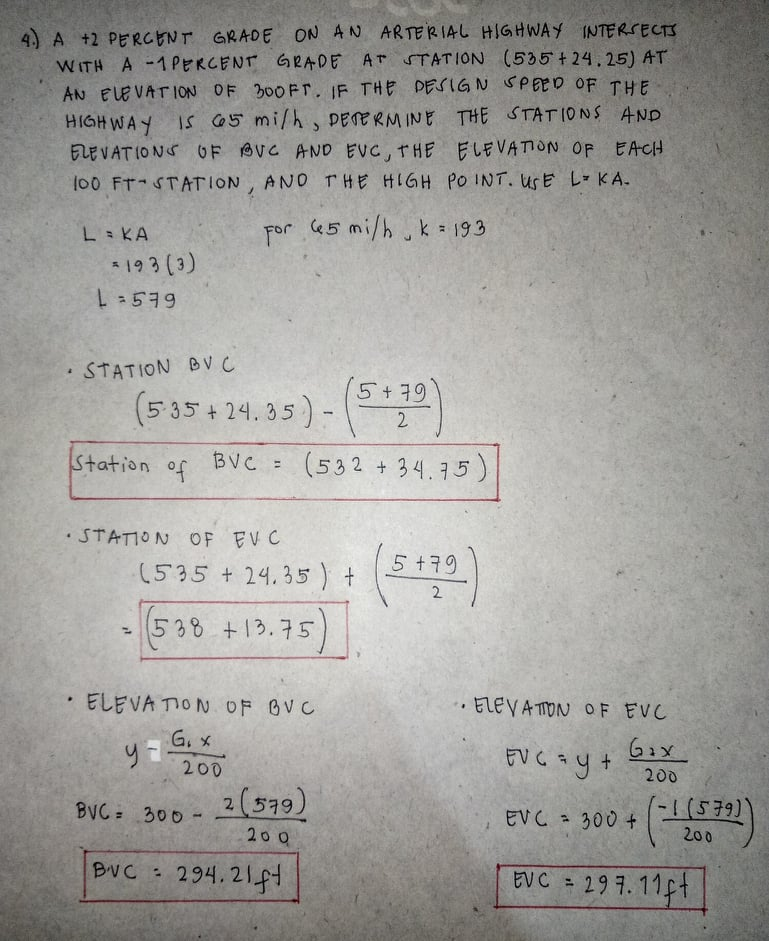Similar Homework Help Questions
• ### highway

A “+4%” grade on an arterial highway intersects with a “-2%” grade at station(214+97.3) at an elevation of 274.3 ft. If the design speed of the highway is 60mph, determine the stations and elevations of the PVC, PVT and the highest pointon the curve. Also, find the elevation at full stations along the curve.

• ### Transportation Problem

A sag vertical curve connects a -1.5%grade and a +1.5% grade on a freight and intercity main linerailway track.If the grades intersect at station (300+25.35)andan elevation of 350.50ft, determine the stations and elevationsof the BVC and EVC and the elevations on the curve at 100-ftintervals.

• ### Problem #3 (20 Points) Vertical Curve Problem (Use both Tangents) See Attached Vertical Curve Drawing Station...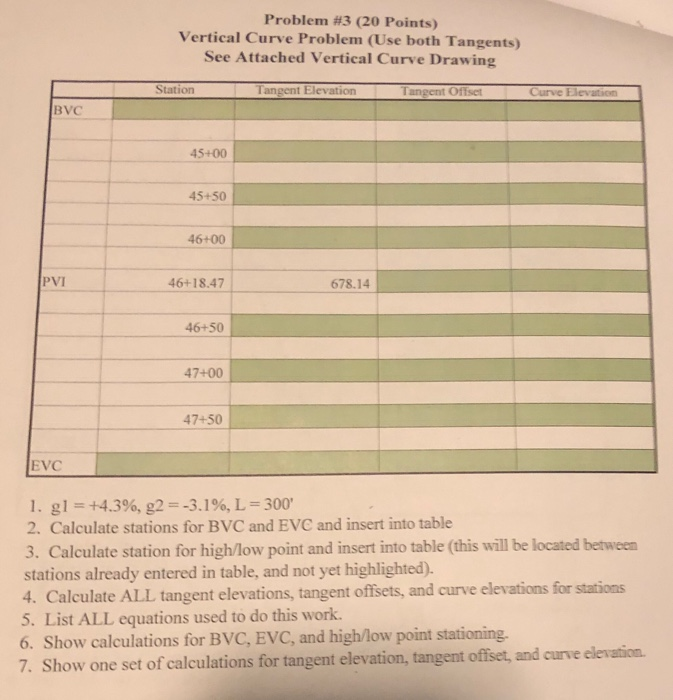Problem #3 (20 Points) Vertical Curve Problem (Use both Tangents) See Attached Vertical Curve Drawing Station Tangent Elevation Tangent Offset В VC 45+00 45+50 46+00 PVI 678.14 46+18.47 46+50 47+00 47+50 EVC I. gl=+4.3%, g2 =-3.196, L = 300, 2. Calculate stations for BVC and EVC and insert into table 3. Calculate station for high/low point and insert into table (this will be located between stations already entered in table, and not yet highlighted). 4. Calculate ALL tangent elevations, tangent...

• ### Transportation Engineering: location, Geometrics, and Drainage

Determine the minimum length of a crest vertical curve if the grades are +4% and -2%, Design speed is 70 mi/h. State assumptions used in solving this problem.

• ### 9:381 LTE Problem 1 A crest vertical curve is to be designed to join a +2% grade with a-2% at a s...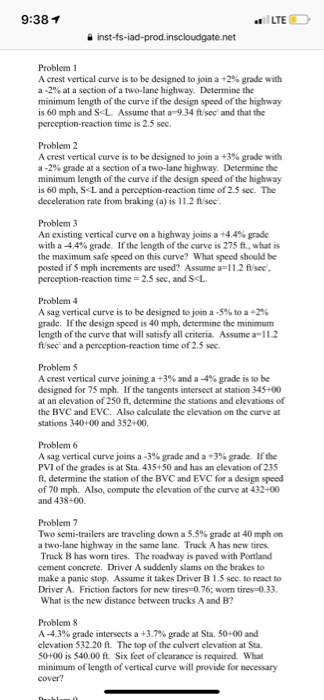9:381 LTE Problem 1 A crest vertical curve is to be designed to join a +2% grade with a-2% at a section of a two-lane highway. Determine the minimum length of the curve if the design speed of the highway is 60 mph and SL. Assume that a-9.34 ftsec' and that the perception-reaction time is 2.5 sec. Problem2 A crest vertical curve is to be designed to join a +3% grade with a-2% grade at a section of a two-lane...

• ### Surveying vertical curves

A vertical curve has a length of 800 ft, g1 = +6%, g2 = -8%. The station and elevation of the PVI is 8+17.36 and 76.85 ft. Assume that there will be stakes placed atthe PVI, BVC, and EVC, the low (or high) point, and at all the 100-ft stations. If the natural ground elevation at the low (or high) point is 76.3 ft, determine thedepth of cut or fill for this point.

• ### 3. A 300 ft vertical curve connects a-2.3% approach grade and a +1.6% departure grade. The PVI is...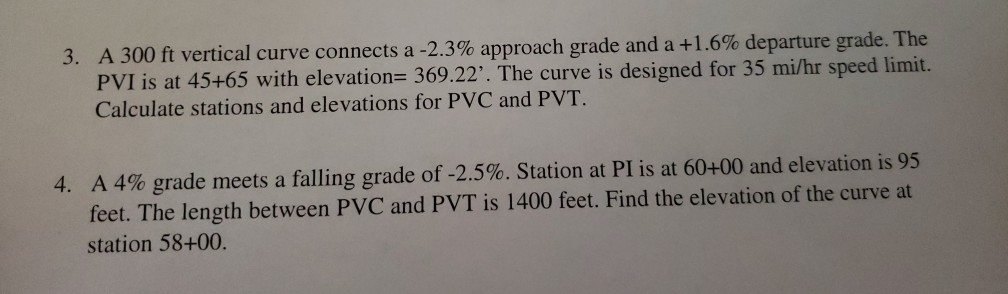3. A 300 ft vertical curve connects a-2.3% approach grade and a +1.6% departure grade. The PVI is at 45+65 with elevation 369.22'. The curve is designed for 35 mi/hr speed limit. Calculate stations and elevations for PVC and PVT A 4% grade meets a failing grade of-25%. Station at P1 is at 60+00 and elevation is 95 feet. The length between PVC and PVT is 1400 feet. Find the elevation of the curve at station 58+00 4. 3. A...

• ### this is a homework for civil engineering " Fundamentals of Transportation Engineering"

A simple highway curve is planned to connect two horizontal tangents that intersect at sta. 2500 + 00.0 at an external angle of 52 degrees.For a designspeed of 60mi/h and a curve radius of about 1.25 times the minimum allowable, calculate:the design rate of superelevationthe required length of superelevation runoff.Assume a four-lane undivided highway and 10-ft lanes, emax=0.10 and fsmax=0.20. (Note that the limiting value of fs from Table 3.5 is usuallysmaller, but use fsmax.)2. Sketch the plan view and...

• ### Transportation Engineering (25 points) As a junior roadway engineer, you have been asked to contr...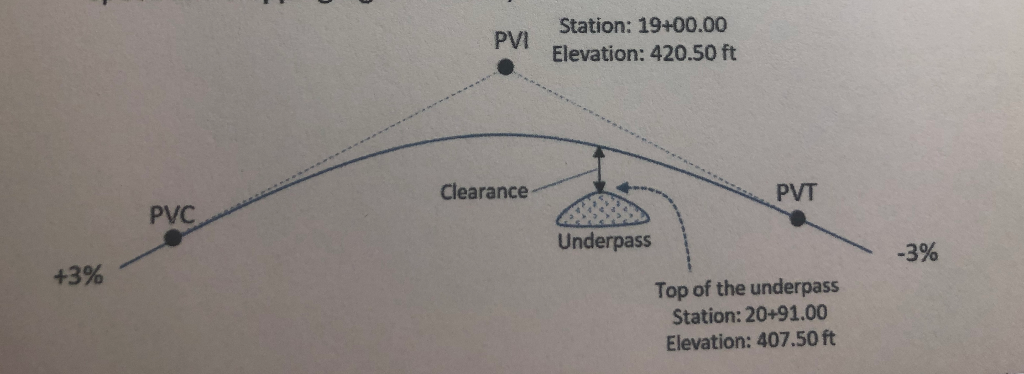Transportation Engineering (25 points) As a junior roadway engineer, you have been asked to contribute to the design of a section of roadway that runs through a national park. An endangered deer population has been identified in the area and a wildlife underpass under a vertical curve has been proposed, as shown in the figure below. An existing underpass is perpendicular to the roadway at station 20+91.00 (not necessarily under the center of the curve). The top of the underpass...

• ### 1) A horizontal curve is being designed around a pond with a tangent length of 1200...

1) A horizontal curve is being designed around a pond with a tangent length of 1200 ft and central angle of 0.5211 radians. If the PI is at station 145+00, determine the station of PT. ⦁   168+45.43 ⦁   156+45.43 ⦁   173+94.00 ⦁   156+72.72 2) A car is traveling downhill on a suburban road with a grade of 3% at a speed of 35 mi/h. Determine the stopping sight distance based on a reaction time of 2.5 second, and a deceleration...

Free Homework App